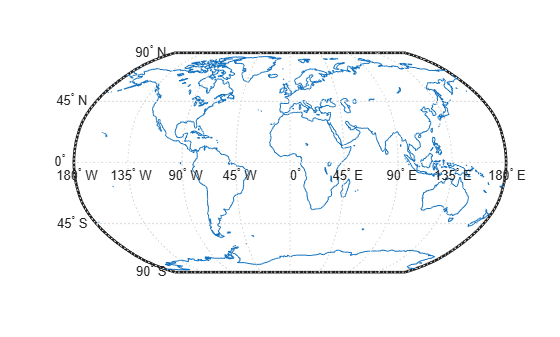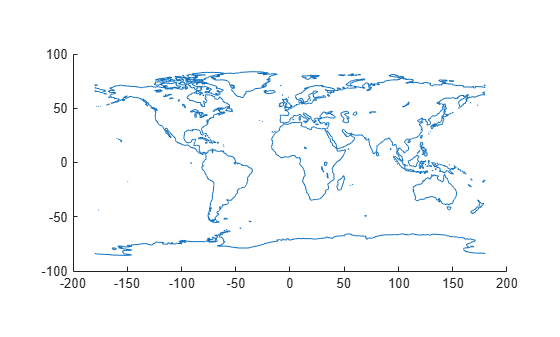# ismap

Determine if axes is `axesm`-based map

## Syntax

``tf = ismap``
``tf = ismap(A)``
``[tf,msg] = ismap(A)``

## Description

example

````tf = ismap` determines if the current axes is an `axesm`-based map. An `axesm`-based map is a map created using the `axesm`, `worldmap`, or `usamap` function. The function returns `1` if the current axes is an `axesm`-based map and `0` otherwise.```
````tf = ismap(A)` determines if the input `A` is an `axesm`-based map.```
````[tf,msg] = ismap(A)` additionally returns the reason why the input is not an `axesm`-based map.```

## Examples

collapse all

Load a MAT file containing the coordinates of global coastlines into the workspace. Create a world map and then plot the coastline data.

```load coastlines figure worldmap("world") geoshow(coastlat,coastlon)```Determine whether the current axes is an `axesm`-based map.

`tf1 = ismap`
```tf1 = 1 ```

Plot the same data without first creating an `axesm`-based map.

```figure geoshow(coastlat,coastlon)```Determine whether the current axes is an `axesm`-based map.

`tf2 = ismap`
```tf2 = 0 ```

## Input Arguments

collapse all

Input array. The array can have any type.

## Output Arguments

collapse all

Indicator for an `axesm`-based map, returned as one of these values:

• `1` indicates that the current axes or `A` is an `axesm`-based map.

• `0` indicates that the current axes or `A` is not an `axesm`-based map.

Data Types: `double`

Reason why the input is not an `axesm`-based map, returned as a character vector. For example, if you specify `A` as a `Line` object, `msg` is ```'Input is not an axes handle.'```.

## Version History

Introduced before R2006a